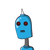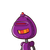# the product of HCF and LCM of two numbers a and b is equal toa) a+bb) a-bc) a x bd) a dividend by b​

the product of HCF and LCM of two numbers a and b is equal to
a) a+b
b) a-b
c) a x b
d) a dividend by b​

### 2 thoughts on “the product of HCF and LCM of two numbers a and b is equal to<br />a) a+b<br />b) a-b<br />c) a x b<br />d) a dividend by b​”

1.The product of HCF and LCM of two numbers a and b is always equal to:

c) a x b

2.